• 关于插值和拟合（出自：https://wenku.baidu.com/view/673980ae580216fc710afd48.html）在实际中，常常要处理由实验或...如果要求这个函数（曲线或曲面）经过所已知的所有的数据点，则称此类问题为插值问题。（不...

关于插值和拟合
（出自：https://wenku.baidu.com/view/673980ae580216fc710afd48.html）
在实际中，常常要处理由实验或测量所得到的一些离散数据。插值与拟合方法就是通过这些数据去确定某一类已知函数的参数或寻求某个近似函数，使所得到的近似函数与已知数据有较高的捏合精度。
如果要求这个函数（曲线或曲面）经过所已知的所有的数据点，则称此类问题为插值问题。（不需要函数表达式）
如果不要求近似函数通过所有数据点，而是要求它能较好地反映数据变化规律的近似函数的方法成为数据拟合。（必须有函数表达式）
近似函数不一定（曲线或曲面）通过所有的数据点。

关于曲线的实现
平滑曲线实现效果图：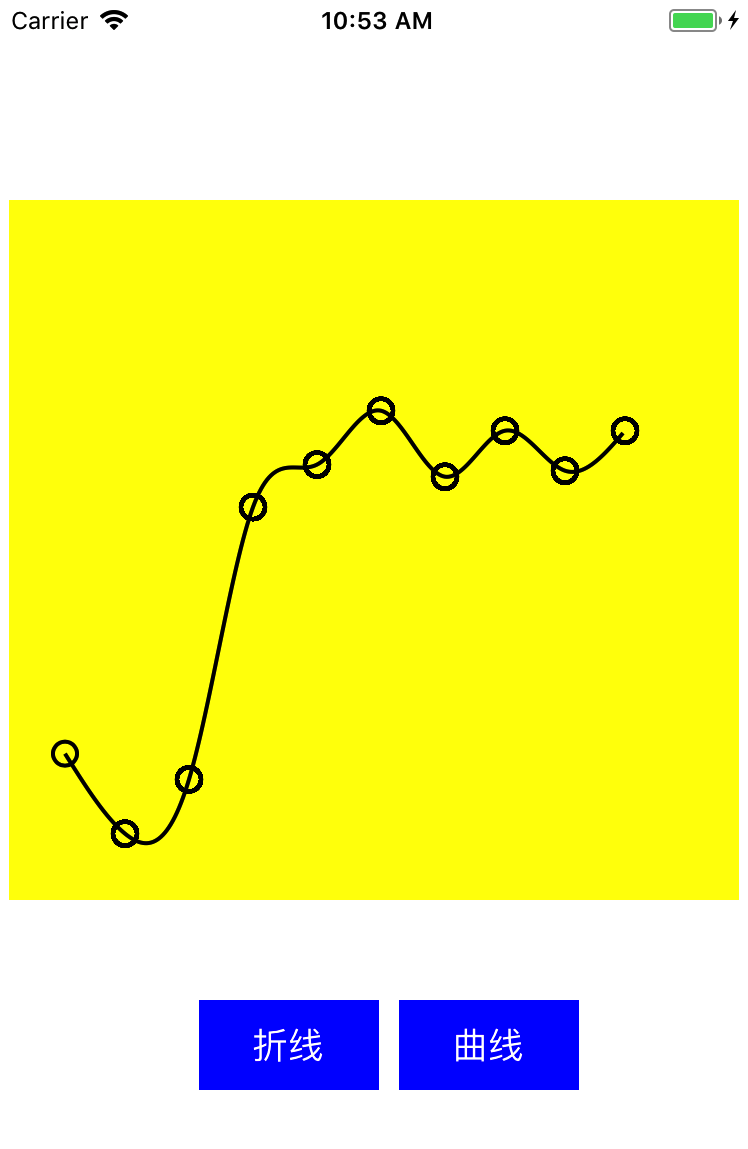由于最终的成品为坐标图表，需要经过已知的数据点，所以平滑曲线的实现采用的插值法（此处采用的三次样条插值法）。
本质上，折线和平滑曲线的实现思路基本是一致的，只不过采用了插值方法在原有点的基础上进行了点的补充，进而无限接近平滑。

线条的绘制为 UIBezierPath + CAShapeLayer 来实现。
再此处有一个渐显的动画，结合 CABasicAnimation  指定 strokeEnd 属性来实现。

三次样条插值参考博客：https://haoxiang.org/2011/06/cubic-spline-interpolation-curve-fitting/


展开全文• plt.scatter绘制散点图 ...使用scipy库可以进行曲线的smooth import matplotlib.pyplot as plt import numpy as np from scipy.interpolate import spline x = np.array([1, 3, 5, 7, 8]) y = np.array([1, 2, 9,...
plt.scatter绘制散点图
plt.plot绘制折线图
使用scipy库可以进行曲线的smooth
import matplotlib.pyplot as plt
import numpy as np
from scipy.interpolate import spline

x = np.array([1, 3, 5, 7, 8])
y = np.array([1, 2, 9, 16, 15])

x_new = np.linspace(x.min(),x.max(),300) #300 represents number of points to make between T.min and T.max
y_smooth = spline(x,y,x_new)
#散点图
plt.scatter(x, y, c='black',alpha = 0.5)  #alpha:透明度) c:颜色
#折线图
plt.plot(x, y, linewidth=1)  #线宽linewidth=1
plt.plot(x, y,c='dimgray', linewidth=0.9,label='示例') #label：某个线条代表什么的标签
plt.legend(loc='best')  # loc选项可以选择label的位置
#平滑后的折线图
plt.plot(x_new,y_smooth,c='red')

# 解决中文显示问题
plt.rcParams['font.sans-serif'] = ['SimHei']  #SimHei黑体  FangSong仿宋
plt.rcParams['axes.unicode_minus'] = False

plt.title("绘图", fontsize=24)#标题及字号
plt.xlabel("X", fontsize=24)#X轴标题及字号
plt.ylabel("Y", fontsize=24)#Y轴标题及字号
plt.tick_params(axis='both', labelsize=14)#刻度大小
#plt.axis([0, 1100, 1, 1100000])#设置坐标轴的取值范围
plt.show()

#plt.save('squares_plot.png'（文件名）, bbox_inches='tight'（将图表多余的空白部分剪掉）)
#用它替换plt.show实现自动保存图表
效果图：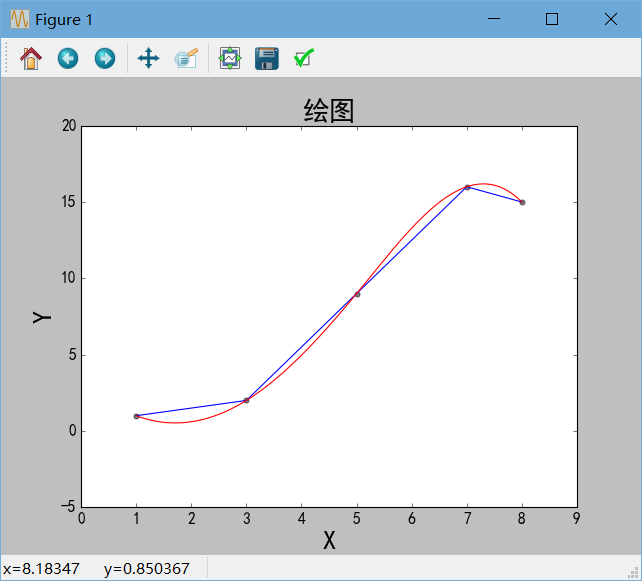关于绘图的颜色c设置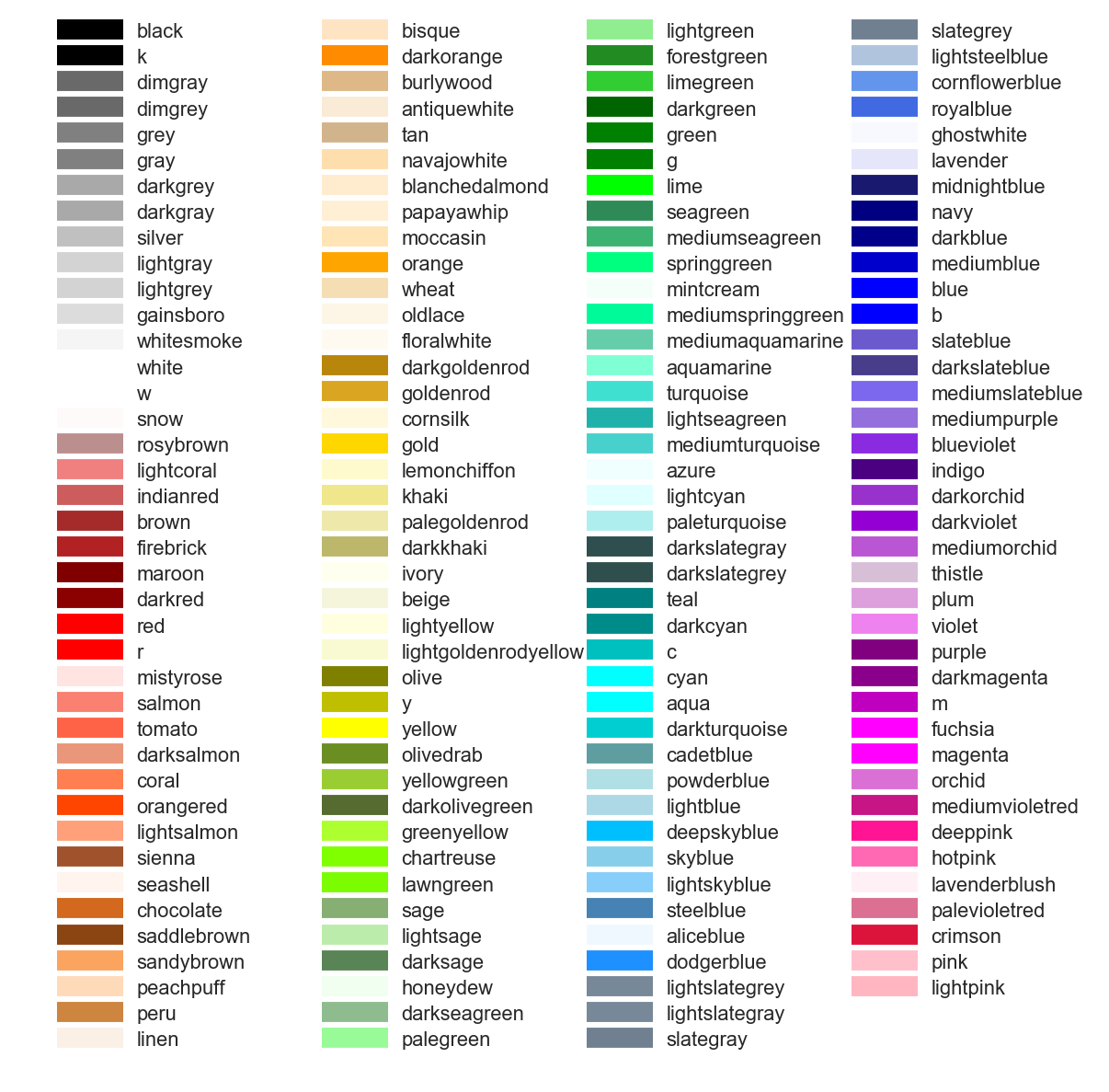展开全文Python matplotlib 教学
• 上一篇文章我们通过getLineChartView来实现了折线...而通过查看API我们发现achartengine还提供了一个getCubeLineChartView来获取一个显示平滑曲线的view，使用起来和获取普通折线图没有太大的区别ChartFactory.getCubeL
上一篇文章我们通过getLineChartView来实现了折线图的显示
ChartFactory.getLineChartView(mContext, dataset, renderer);
而通过查看API我们发现achartengine还提供了一个getCubeLineChartView来获取一个显示平滑曲线的view，使用起来和获取普通折线图没有太大的区别
ChartFactory.getCubeLineChartView(mContext, dataset, renderer, 0.3F);
这里多了最后一个设置平滑度的系数，效果图如下：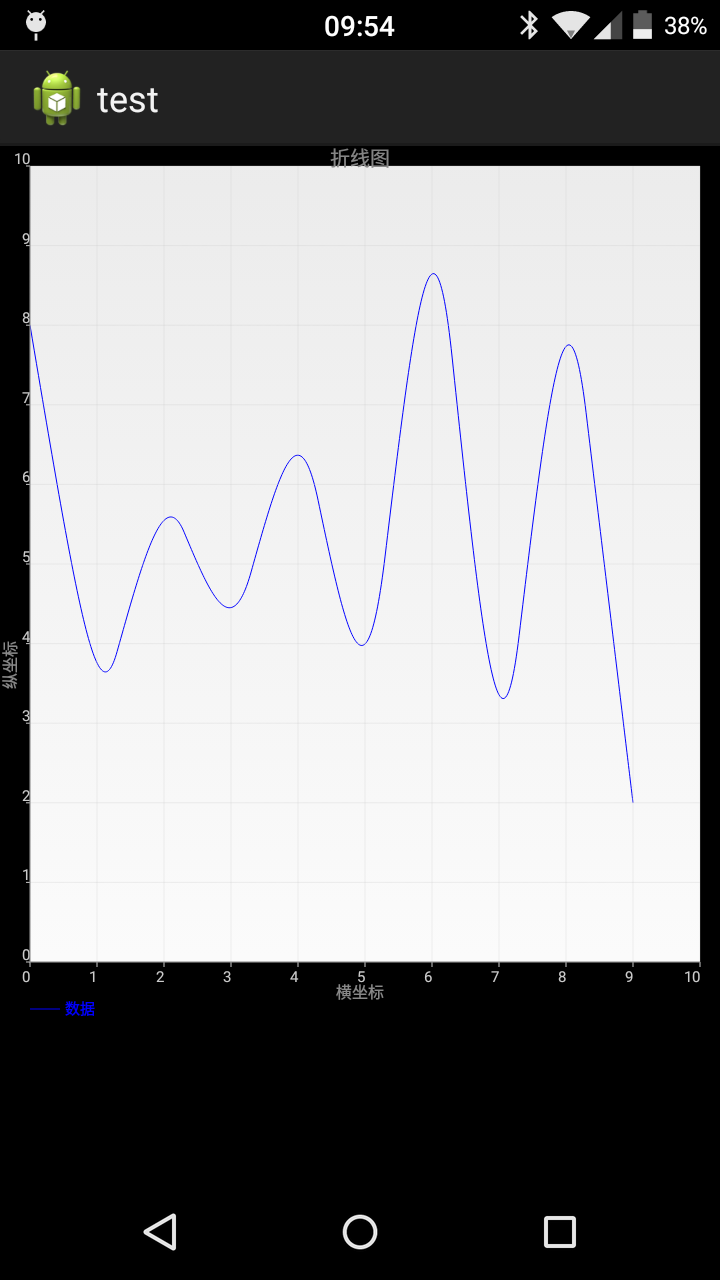通过观察效果图我们发现曲线并没有经过我们设置的点，怎么回事，我们的程序有BUG了？显然并不是。在解释这个情况之前，我们需要理解一个概念：贝塞尔曲线
这里我假设你已经大概浏览了维基百科的内容，因此我们知道贝塞尔曲线必过两个端点，并且受控制点控制，achartengine在绘制平滑曲线的时候使用的三阶贝塞尔曲线（安卓在Path.java这个类中只提供了二阶quadTo和三阶cubicTo贝塞尔曲线），这里引用一下维基百科上的图片可以更加直观的展现三阶贝塞尔曲线的绘制过程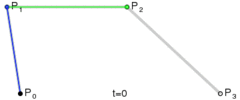通过观察上图我们发现三阶贝塞尔曲线必过P0和P3点，受P1和P2点控制，好了概念介绍完毕，我们就可以来解释一下为什么achartengine的平滑曲线没有经过数据点了。
在achartengine的源码CubicLineChart.java中
  protected void drawPath(Canvas canvas, List<Float> points, Paint paint, boolean circular) {
Path p = new Path();
float x = points.get(0);
float y = points.get(1);
p.moveTo(x, y);

int length = points.size();
if (circular) {
length -= 4;
}

Point p1 = new Point();
Point p2 = new Point();
Point p3 = new Point();
for (int i = 0; i < length; i += 2) {
int nextIndex = i + 2 < length ? i + 2 : i;
int nextNextIndex = i + 4 < length ? i + 4 : nextIndex;
calc(points, p1, i, nextIndex, mSecondMultiplier);
p2.setX(points.get(nextIndex));
p2.setY(points.get(nextIndex + 1));
calc(points, p3, nextIndex, nextNextIndex, mFirstMultiplier);
// From last point, approaching x1/y1 and x2/y2 and ends up at x3/y3
p.cubicTo(p1.getX(), p1.getY(), p2.getX(), p2.getY(), p3.getX(), p3.getY());
}
...//省略部分代码
canvas.drawPath(p, paint);
}
其中P对应上图中的P0，而P1,P2,P3依次对应贝塞尔曲线的P1,P2,P3，这里需要说明一下的是，P1和P3是根据平滑度系数计算得出的点，而P2则是我们自己设置的点，根据贝塞尔曲线公式，曲线是不会经过P2的，因为我们的数据点在贝塞尔曲线中充当了控制点的角色。这就解释了为什么我们使用getCubeLineChartView的时候曲线没有经过数据点。  有些聪明的同学可能会立即反应，如果我们将数据点设置为曲线的端点就可以让曲线经过数据点了，因为贝塞尔曲线是必过端点的，这里我不建议这样做，因为这样绘制出来的曲线会非常难看，喜欢动手的同学可以自己试一下
展开全文• 程序为MFC对话框程序 包括 三次样条插值的计算类，自己封的很好用。 贝塞尔曲线 GDI+平滑曲线 用这三种算法来平滑曲线，在程序中可以很值观的看出来，自己写的，给点辛苦钱吧。
• 基于刘大师的自定义自定义控件之简单曲线图表控件，添加了动画和曲线平滑的功能。 动画就是数据点有个滑动渐变的过程。 曲线平滑主要采用贝塞尔曲线产生平滑曲线。 二.功能演示 三.部分代码说明 1.平滑曲线生成 ...
一.说明：
基于刘大师的自定义自定义控件之简单曲线图表控件，添加了动画和曲线平滑的功能。 动画就是数据点有个滑动渐变的过程。 曲线平滑主要采用贝塞尔曲线产生平滑曲线。
二.功能演示三.部分代码说明
1.平滑曲线生成
QPainterPath CurveChart::generateSmoothCurve(const QList<QPointF> &points)
{
QPainterPath path;
int len = points.size();
if (len < 2) {
return path;
}

QList<QPointF> firstControlPoints;
QList<QPointF> secondControlPoints;

calculateControlPoints(points, &firstControlPoints, &secondControlPoints);
path.moveTo(points.x(), points.y());

// Using bezier curve to generate a smooth curve.
for (int i = 0; i < len - 1; ++i) {
path.cubicTo(firstControlPoints[i], secondControlPoints[i], points[i+1]);
}

return path;
}

void CurveChart::calculateControlPoints(const QList<QPointF> &knots, QList<QPointF> *firstControlPoints, QList<QPointF> *secondControlPoints)
{
int n = knots.size() - 1;
for (int i = 0; i < n; ++i) {
firstControlPoints->append(QPointF());
secondControlPoints->append(QPointF());
}

if (n == 1) {
// Special case: Bezier curve should be a straight line.
// P1 = (2P0 + P3) / 3
(*firstControlPoints).rx() = (2 * knots.x() + knots.x()) / 3;
(*firstControlPoints).ry() = (2 * knots.y() + knots.y()) / 3;
// P2 = 2P1 – P0
(*secondControlPoints).rx() = 2 * (*firstControlPoints).x() - knots.x();
(*secondControlPoints).ry() = 2 * (*firstControlPoints).y() - knots.y();
return;
}

// Calculate first Bezier control points
double *xs = new double[n];
double *ys = new double[n];
double *rhsx = new double[n]; // Right hand side vector
double *rhsy = new double[n]; // Right hand side vector

// Set right hand side values
for (int i = 1; i < n - 1; ++i) {
rhsx[i] = 4 * knots[i].x() + 2 * knots[i + 1].x();
rhsy[i] = 4 * knots[i].y() + 2 * knots[i + 1].y();
}
rhsx = knots.x() + 2 * knots.x();
rhsx[n - 1] = (8 * knots[n - 1].x() + knots[n].x()) / 2.0;
rhsy = knots.y() + 2 * knots.y();
rhsy[n - 1] = (8 * knots[n - 1].y() + knots[n].y()) / 2.0;

// Calculate first control points coordinates
calculateFirstControlPoints(xs, rhsx, n);
calculateFirstControlPoints(ys, rhsy, n);

// Fill output control points.
for (int i = 0; i < n; ++i) {
(*firstControlPoints)[i].rx() = xs[i];
(*firstControlPoints)[i].ry() = ys[i];
if (i < n - 1) {
(*secondControlPoints)[i].rx() = 2 * knots[i + 1].x() - xs[i + 1];
(*secondControlPoints)[i].ry() = 2 * knots[i + 1].y() - ys[i + 1];
} else {
(*secondControlPoints)[i].rx() = (knots[n].x() + xs[n - 1]) / 2;
(*secondControlPoints)[i].ry() = (knots[n].y() + ys[n - 1]) / 2;
}
}

delete[] xs;
delete[] ys;
delete[] rhsx;
delete[] rhsy;
}

void CurveChart::calculateFirstControlPoints(double *&result, const double *rhs, int n)
{
double *tmp = new double[n];
double b = 2.0;
result = rhs / b;

// Decomposition and forward substitution.
for (int i = 1; i < n; i++) {
tmp[i] = 1 / b;
b = (i < n - 1 ? 4.0 : 3.5) - tmp[i];
result[i] = (rhs[i] - result[i - 1]) / b;
}

for (int i = 1; i < n; i++) {
result[n - i - 1] -= tmp[n - i] * result[n - i]; // Backsubstitution.
}

delete[] tmp;
}

只需调用generateSmoothCurve，将原始的点输入进去返回QPainterPath，然后再用painter调drawPath就行了，比如painter->drawPath(generateSmoothCurve(listPoints)); （ps:这部分的代码抄的深度系统监视器的源码）
2.动画说明 就是一个渐变的过程，比如第二个点来时，原本的第一个位置的点要慢慢移动到第二个点，以此循环，直到所有的点都移动完成，基本原理就是开个定时器，数据改变时每隔1ms调用1次update，每次update时都从上次的点逐渐往目标逼近1个像素点，直至重合的过程。
展开全文• 1.双折线图（平滑曲线），展现对比效果 2.X轴单位，默认显示在1（月） 3.Y轴单位（%）或者其他,但文字写上去总是有点丑，就没放在Y轴 4.MarkView这里的不同点在于，点击一个点显示相同X轴对比的数据 5.新版...
• 平滑曲线是所有涉及到曲线图的项目中，绕不开的一个话题，尽管很多人爱看折线图，但是很多时候来个平滑曲线图，会更加赏心悦目，这就好比现在的手机app移动客户端上，从最初的四方四正到现在的平滑圆角大行其道，...Qt可视化
• 今天写机械设计的带传动实验报告时，需要绘制滑动曲线ε-F和效率曲线η-F的关系曲线——正在学MATLAB的我内心狂喜，直接复制表格中的数据，掏出了plot函数。>> S=[0.348.55.50.364.64.80.380.630.780.63.34....
• 背景 公司最近引入了 Flutter 技术栈，Flutter 是谷歌的移动 UI ...比如项目中需要用到图表 UI 组件，经过一番调研，Google/charts 功能最强大，样式最丰富（详见 online gallery），于是引入到项目中。但是 chart...
• 之前有写过一个图表lib，但是开发的速度，大多很难跟上产品需求变化的脚步，所以修改了下原先的图表库，支持图表下面能整合table显示对应的类目，用曲线替换了折线，支持多曲线的显示，增加了显示的动画，，增加了...
• canvas画出来的线条存在锯齿，不够平滑。这篇文章主要介绍了canvas进阶之如何画出平滑曲线，小编觉得挺不错的，现在分享给大家，也给大家做个参考。一起跟随小编过来看看吧
• ## C++ 曲线平滑

千次阅读 2019-08-18 15:15:29
直接展示出来的效果不是很好，故在网上查找了很久曲线平滑的方法，最终找到了了一套比较适合的方法，思路比较简单，对于现在的项目来说效果也还不错，故记录一下。 原始数据大小分别为881、490和1711，直接展示如下...C++
• 曲线拟合也称为曲线逼近，它只要求拟合的曲线合理的反应数据的基本趋势，而并不要求曲线一定经过数据点，只是要求在整体上“尽量好”的逼近原函数。这时,在每个已知点上就会有误差,数据拟合就是从整体...
• MATLAB中自带的cftool拟合工具箱不能将多条曲线同时画在同一副图中，而常规的plot()函数又不能拟合平滑直线，接下来总结一种可以利用cftool导出的代码，在一张图中拟合多条平滑曲线。 首先输入所要拟合的数据，如x,...
• 首先给出一个没有smooth过的曲线import xlrd import numpy as np import matplotlib.pyplot as plt from scipy.interpolate import spline workbook = xlrd.open_workbook("/Users/lm/Documents/实验一/算法...
• 目录 利用绘制图表：​​​​​​matplotlib官网 1. 小试牛刀——柱状图 1.1 matplotlib库默认英文字体 2. 折线图绘制 2.1 读取exal方法 2.1.1 数据处理常用库：pandas 2.1.2 找到pandas在pathon安装的位置Lib->...python 机器学习
• 详细注解代码里面有，就不一一说明了，说下需要注意的事项： renderer.setPanEnabled(true, false);// 设置横坐标可以滑动，纵坐标不可以 renderer.setZoomEnabled(false, false);...圆滑单曲线： /** * 圆滑
• import matplotlib.pyplot as plt import numpy as np from scipy....plt.show() #plt.save('squares_plot.png'（文件名）, bbox_inches='tight'（将图表多余的空白部分剪掉）) #用它替换plt.show实现自动保存图表
• 众所周知想用canvas画一条曲线我们可以使用这些函数： 二次曲线：quadraticCurveTo(cp1x, cp1y, x, y) ...但是如果一组点给你，怎么通过这些已知点画一条平滑曲线呢？使用二次曲线，或是圆弧？恐怕这些都...
• 1.HTML5 Canvas动画折线图 可动态...第二个是这个HTML5折线图表可以让你动态添加数据节点，并且用折线平滑地连接，Canvas是这款折线图的一个画板。2.基于HTML5 Canvas的图表插件Chart.js2 . chart.js是一款基于HTM...
• 利用Excel进行指数平滑分析与预测(2)【例】连续10年的灌溉面积。第一步，录入数据(图1)。图1 原始数据第二步，选项设置。沿着主菜单的“工具(T)→数据分析(D)”路径打开“数据分析”选项框，选中“指数平滑”(图2)。...
• 主要的命令格式如下： (1)plot(x,’Name’,Value) x表示绘制图表的数据，Name表示属性的字符选项，Value表示相应的选值。当x是一维数组时，以数组元素下标为横坐标，元素数值为纵坐标绘制曲线；当x是二维数组时，...
• //设置精度，大概意思是在源数据两个点之间插入5个点以拟合出一条平滑曲线 splinerenderer.setSeriesStroke(0, new BasicStroke(4.0F, 1, 1, 1.0F)); // splinerenderer.setSeriesStroke(1, new ...java 分类
• 2、平滑曲线 3、动画加载 4、图例 5、标记线（最大、最小、平均值、自定义值等） 6、标定位置（最大、最小、平均值、自定义值等） 7、范围蒙版（渐变色/单色） 8、线条渐变色 9、线条点显示在刻度中间位置 ...
• 求Excel图表曲线上任意点的坐标.rar,在许多场合中经常有这样的问题：已知一组数据，并据此绘制了曲线图，如何求曲线上任意一点的坐标。如本例所示，如何根据已知的Y值，求X值。Excel应用...